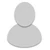## FANDOM

1,629 Pages

• I ignored "x" obviously.

• Wait what?

-checks- oh yeah...I can't even remember doing that.

• I assumed it was common log(2) x 6200, as the two was not in subscript, but you could well be right.

• ...wat. My schooling has not prepared me for this.

• In case you were curious, logs are basically just another way of writing exponents, but so that the exponent is the solution. Saying logab=c means that ac=b. If a is not given, it is assumed to be 10.

Assuming I was correct in the assumption that Lysy intended "(log102)*6400," not "log26400," "log2=x" is the same as saying 10x=2, and x=about 0.301, which is of course only one part of Lysy's damage.

• ...thanks.

• It takes a bit of fenagling. What Catfish meant was that a logarithm is the inverse of an exponential function, a way to solve it. Think about bx=y, and then think about the inverse (where x and y are switched): by=x. How do you solve for y? Logs are the way people came up with to do so.

• Last term in Maths we looked at similarities between patterns.

•